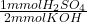## You have 26.7 mL of 0.061 mol/L aqueous potassium hydroxide (KOH(aq)) in a conical flask. In a burette you have 0.086 mol/L H2SO4(aq).

Question

You have 26.7 mL of 0.061 mol/L aqueous potassium hydroxide (KOH(aq)) in a conical flask. In a burette
you have 0.086 mol/L H2SO4(aq). If you titrate the two solutions, what volume (mL) of the H2SO4 solution
is required to reach the equivalence point (endpoint)?

in progress 0
5 months 2021-08-30T10:24:41+00:00 1 Answers 2 views 0

## Answers ( )

9.47 mL

Explanation:

The reaction that takes place is:

• 2KOH + H₂SO₄ → K₂SO₄ + 2H₂O

First we calculate how many KOH moles reacted, using the given concentration and volume of KOH solution:

• 0.061 mol/L = 0.061 mmol/mL
• 0.061 mmol/mL * 26.7 mL = 1.6287 mmol KOH

Then we convert KOH moles into H₂SO₄ moles, using the stoichiometric coefficients:

• 1.6287 mmol KOH *= 0.8144 mmol H₂SO₄

Finally we calculate the required volume of the H₂SO₄ solution, using the number of moles and given concentration:

• 0.8144 mmol ÷ 0.086 mmol/mL = 9.47 mL